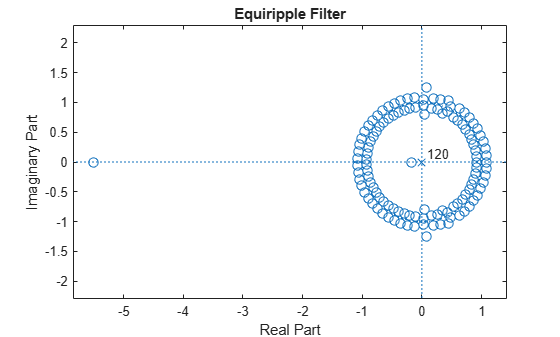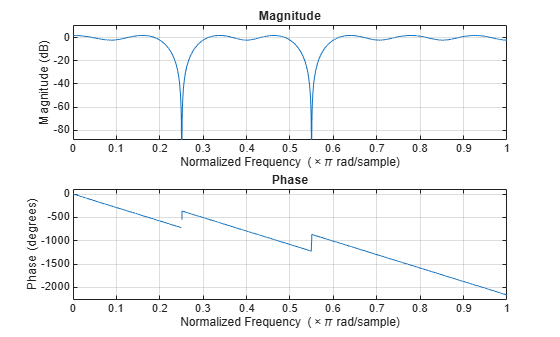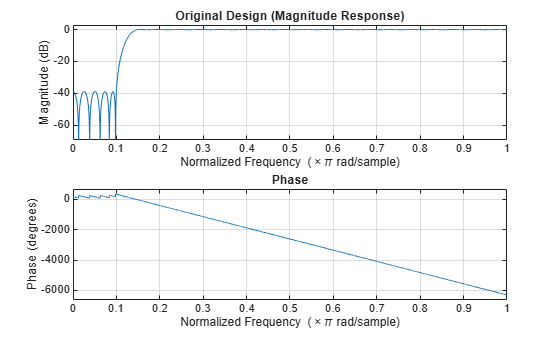Documentation

### This is machine translation

Mouseover text to see original. Click the button below to return to the English version of the page.

# fdesign.arbmag

Arbitrary response magnitude filter specification object

## Syntax

```D= fdesign.arbmag D= fdesign.arbmag(SPEC) D = fdesign.arbmag(SPEC,specvalue1,specvalue2,...) D = fdesign.arbmag(specvalue1,specvalue2,specvalue3) D = fdesign.arbmag(...,Fs) ```

## Description

`D= fdesign.arbmag` constructs an arbitrary magnitude filter specification object `D`.

`D= fdesign.arbmag(SPEC)` initializes the `Specification` property to `SPEC`. The input argument `SPEC` must be one of the entries shown in the following table. Specification entries are not case sensitive.

### Note

Specification entries marked with an asterisk require the DSP System Toolbox™ software.

• `'N,F,A'` — Single band design (default)

• `'F,A,R'` — Single band minimum order design *

• `'N,B,F,A'` — Multiband design

• `'N,B,F,A,C'` — Constrained multiband design *

• `'B,F,A,R'` — Multiband minimum order design *

• `'Nb,Na,F,A'` — Single band design *

• `'Nb,Na,B,F,A'` — Multiband design *

The `SPEC` entries are defined as follows:

• `A` — Amplitude vector. Values in `A` define the filter amplitude at frequency points you specify in `f`, the frequency vector. If you use `A`, you must use `F` as well. Amplitude values must be real. For complex values designs, use `fdesign.arbmagnphase`.

• `B` — Number of bands in the multiband filter

• `C` — Constrained band flag. This enables you to constrain the passband ripple in your multiband design. You cannot constrain the passband ripple in all bands simultaneously.

• `F` — Frequency vector. Frequency values in specified in `F` indicate locations where you provide specific filter response amplitudes. When you provide `F`, you must also provide `A`.

• `N` — Filter order for FIR filters and the numerator and denominator orders for IIR filters.

• `Nb` — Numerator order for IIR filters

• `Na` — Denominator order for IIR filter designs

• `R` — Ripple

By default, this method assumes that all frequency specifications are supplied in normalized frequency.

### Specifying Frequency and Amplitude Vectors

`F` and `A` are the input arguments you use to define the filter response desired. Each frequency value you specify in `F` must have a corresponding response value in `A`. The following table shows how `F` and `A` are related.

Define the frequency vector `F` as ```[0 0.25 0.3 0.4 0.5 0.6 0.7 0.75 1.0]```

Define the response vector `A` as ```[1 1 0 0 0 0 0 1 1]```

These specifications connect `F` and `A` as shown here:

F (Normalized Frequency)

A (Response Desired at F)

0

1

0.25

1

0.3

0

0.4

0

0.5

0

0.6

0

0.7

0

0.75

1

1.0

1

Different specifications can have different design methods available. Use `designmethods` to get a list of design methods available for a given specification and filter specification object.

Use `designopts` to get a list of design options available for a filter specification object and a given design method. Enter `help(D,METHOD)` to get detailed help on the available design options for a given design method.

`D = fdesign.arbmag(SPEC,specvalue1,specvalue2,...)` initializes the specifications with `specvalue1`, `specvalue2`. Use `get(D,'Description')` for descriptions of the various specifications `specvalue1`, `specvalue2`, ... `specvalueN`.

`D = fdesign.arbmag(specvalue1,specvalue2,specvalue3)` uses the default specification `'N,F,A'`, setting the filter order, filter frequency vector, and the amplitude vector to the values `specvalue1`, `specvalue2`, and `specvalue3`.

`D = fdesign.arbmag(...,Fs)` specifies the sampling frequency in Hz. All other frequency specifications are also assumed to be in Hz when you specify `Fs`.

## Examples

collapse all

Use `fdesign.arbmag` to design a three-band filter.

• Define the frequency vector `F` = [0 0.25 0.3 0.4 0.5 0.6 0.7 0.75 1.0].

• Define the response vector `A` = [1 1 0 0 0 0 0 1 1].

```N = 150; B = 3; F = [0 .25 .3 .4 .5 .6 .7 .75 1]; A = [1 1 0 0 0 0 0 1 1]; A1 = A(1:2); A2 = A(3:7); A3 = A(8:end); F1 = F(1:2); F2 = F(3:7); F3 = F(8:end); d = fdesign.arbmag('N,B,F,A',N,B,F1,A1,F2,A2,F3,A3); Hd = design(d); fvtool(Hd)```A response with two passbands -- one roughly between 0 and 0.25 and the second between 0.75 and 1 -- results from the mapping between `F` and `A`.

Use `fdesign.arbmag` to design a single band equiripple filter.

Specify 100 frequency points.

```n = 120; f = linspace(0,1,100); as = ones(1,100)-f*0.2; absorb = [ones(1,30),1-0.6*bohmanwin(10)',ones(1,5), ... 1-0.5*bohmanwin(8)',ones(1,47)]; a = as.*absorb; d = fdesign.arbmag('N,F,A',n,f,a); hd1 = design(d,'equiripple');```

Design a minimum-phase equiripple filter. Visualize the poles and zeros of the two filters.

```hd2 = design(d,'equiripple','MinPhase',true); hfvt = fvtool(hd1,hd2,'Analysis','polezero'); legend(hfvt,'Equiripple Filter','Minimum-phase Equiripple Filter')```Use `fdesign.arbmag` to design a multiband minimum order filter.

Place the notches at $0.25\pi$ and $0.55\pi$ rad/sample.

```d = fdesign.arbmag('B,F,A,R'); d.NBands = 5; d.B1Frequencies = [0 0.2]; d.B1Amplitudes = [1 1]; d.B1Ripple = 0.25; d.B2Frequencies = 0.25; d.B2Amplitudes = 0; d.B3Frequencies = [0.3 0.5]; d.B3Amplitudes = [1 1]; d.B3Ripple = 0.25; d.B4Frequencies = 0.55; d.B4Amplitudes = 0; d.B5Frequencies = [0.6 1]; d.B5Amplitudes = [1 1]; d.B5Ripple = 0.25; Hd = design(d,'equiripple');```

Visualize the frequency response of the resulting filter.

`fvtool(Hd)`Use `fdesign.arbmag` to design a multiband constrained FIR filter.

Force the frequency response at $0.15\pi$ rad/sample to 0 dB.

```d = fdesign.arbmag('N,B,F,A,C',82,2); d.B1Frequencies = [0 0.06 0.1]; d.B1Amplitudes = [0 0 0]; d.B2Frequencies = [0.15 1]; d.B2Amplitudes = [1 1];```

Design a filter with no constraints.

`Hd1 = design(d,'equiripple','B2ForcedFrequencyPoints',0.15);`

Add a constraint to the first band to increase attenuation.

```d.B1Constrained = true; d.B1Ripple = 0.001; Hd2 = design(d,'equiripple','B2ForcedFrequencyPoints',0.15);```

Visualize the frequency response.

```hfvt = fvtool(Hd1,Hd2); legend(hfvt,'Original Design','Design with Constrained Stopband Ripple')```